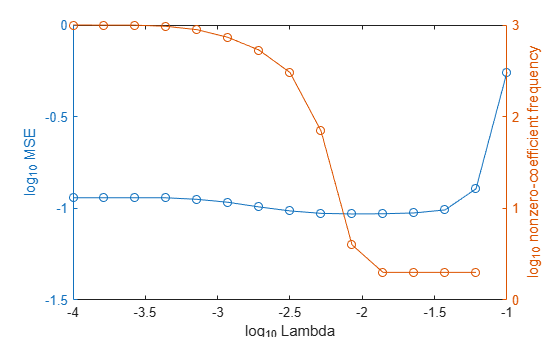selectModels

Class: RegressionLinear

Select fitted regularized linear regression models

Description

example

SubMdl = selectModels(Mdl,idx) returns a subset of trained linear regression models from a set of linear regression models (Mdl) trained using various regularization strengths. The indices idx correspond to the regularization strengths in Mdl.Lambda, and specify which models to return.

Input Arguments

expand all

Linear regression models trained using various regularization strengths, specified as a RegressionLinear model object. You can create a RegressionLinear model object using fitrlinear.

Although Mdl is one model object, if numel(Mdl.Lambda) = L ≥ 2, then you can think of Mdl as L trained models.

Indices corresponding to regularization strengths, specified as a numeric vector of positive integers. Values of idx must be in the interval [1,L], where L = numel(Mdl.Lambda).

Data Types: double | single

Output Arguments

expand all

Subset of linear regression models trained using various regularization strengths, returned as a RegressionLinear model object.

Examples

expand all

Simulate 10000 observations from this model

$y={x}_{100}+2{x}_{200}+e.$

• $X=\left\{{x}_{1},...,{x}_{1000}\right\}$ is a 10000-by-1000 sparse matrix with 10% nonzero standard normal elements.

• e is random normal error with mean 0 and standard deviation 0.3.

rng(1) % For reproducibility
n = 1e4;
d = 1e3;
nz = 0.1;
X = sprandn(n,d,nz);
Y = X(:,100) + 2*X(:,200) + 0.3*randn(n,1);

Create a set of 15 logarithmically-spaced regularization strengths from $1{0}^{-4}$ through $1{0}^{-1}$.

Lambda = logspace(-4,-1,15);

Hold out 30% of the data for testing. Identify the test-sample indices.

cvp = cvpartition(numel(Y),'Holdout',0.30);
idxTest = test(cvp);

Train a linear regression model using lasso penalties with the strengths in Lambda. Specify the regularization strengths, optimizing the objective function using SpaRSA, and the data partition. To increase execution speed, transpose the predictor data and specify that the observations are in columns.

X = X';
CVMdl = fitrlinear(X,Y,'ObservationsIn','columns','Lambda',Lambda,...
'Solver','sparsa','Regularization','lasso','CVPartition',cvp);
Mdl1 = CVMdl.Trained{1};
numel(Mdl1.Lambda)
ans = 15

Mdl1 is a RegressionLinear model. Because Lambda is a 15-dimensional vector of regularization strengths, you can think of Mdl1 as 15 trained models, one for each regularization strength.

Estimate the test-sample mean squared error for each regularized model.

mse = loss(Mdl1,X(:,idxTest),Y(idxTest),'ObservationsIn','columns');

Higher values of Lambda lead to predictor variable sparsity, which is a good quality of a regression model. Retrain the model using the entire data set and all options used previously, except the data-partition specification. Determine the number of nonzero coefficients per model.

Mdl = fitrlinear(X,Y,'ObservationsIn','columns','Lambda',Lambda,...
'Solver','sparsa','Regularization','lasso');
numNZCoeff = sum(Mdl.Beta~=0);

In the same figure, plot the MSE and frequency of nonzero coefficients for each regularization strength. Plot all variables on the log scale.

figure;
[h,hL1,hL2] = plotyy(log10(Lambda),log10(mse),...
log10(Lambda),log10(numNZCoeff));
hL1.Marker = 'o';
hL2.Marker = 'o';
ylabel(h(1),'log_{10} MSE')
ylabel(h(2),'log_{10} nonzero-coefficient frequency')
xlabel('log_{10} Lambda')
hold offSelect the index or indices of Lambda that balance minimal classification error and predictor-variable sparsity (for example, Lambda(11)).

idx = 11;
MdlFinal = selectModels(Mdl,idx);

MdlFinal is a trained RegressionLinear model object that uses Lambda(11) as a regularization strength.

Tips

One way to build several predictive linear regression models is:

1. Hold out a portion of the data for testing.

2. Train a linear regression model using fitrlinear. Specify a grid of regularization strengths using the 'Lambda' name-value pair argument and supply the training data. fitrlinear returns one RegressionLinear model object, but it contains a model for each regularization strength.

3. To determine the quality of each regularized model, pass the returned model object and the held-out data to, for example, loss.

4. Identify the indices (idx) of a satisfactory subset of regularized models, and then pass the returned model and the indices to selectModels. selectModels returns one RegressionLinear model object, but it contains numel(idx) regularized models.

5. To predict class labels for new data, pass the data and the subset of regularized models to predict.